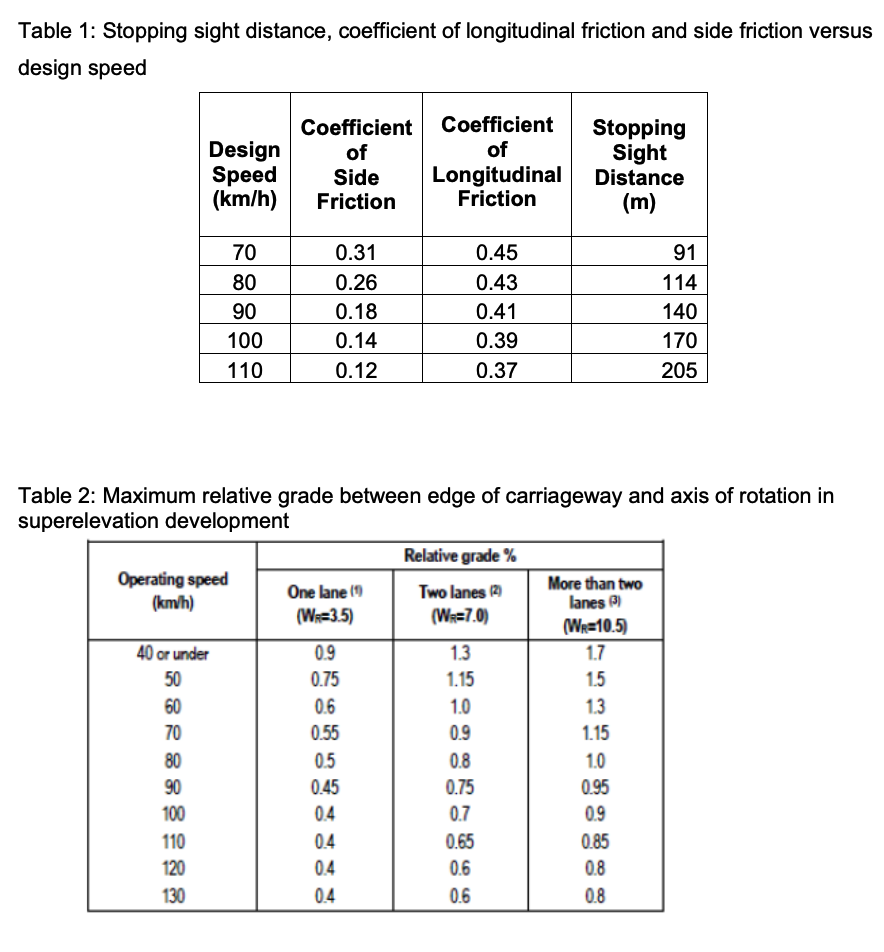### Design Speed

Design Speed

The maximum safe speed of vehicles assumed for a geometrical design of a highway is known as design speed.

The design speed is the most important factor controlling the geometric design elements of highways, the design speed is decided considering the overall requirements of highways.

The design speed dependent upon the following factors:

1. Types and conditions of the road surface.

3. Nature and intensity of traffic.

4. Types of the curve along the road.

5. Sight distance required.

6. Nature of the country.

• BRAKING EFFICIENCY

Braking efficiency, now the word efficiency means how much efficiency of any system or machine or anything is related to its job or output to be given. The same thing can be related to brakes of an automobile. So we can say that braking efficiency indicates to us how reliable or efficient are the brakes in doing the job of stopping our vehicles. This is indicated by the percentage of brake force for the vehicle weight.

So the formula for the braking efficiency can be given as :

Braking efficiency = (brake force / vehicle weight) * 100

This value is always less than hundred as it is never possible to get 100 % tyre adhesion to the road.

The concept of braking efficiency can be related to coefficient of friction as coefficient of friction is ratio of friction force to the normal load. Here frictional force is provided as brake force and the normal load is the vehicle load.

• Frictional resistance between road and tyre

1. The friction developed between road and tire depends upon coefficient of friction.

2. Higher is coefficient of friction, higher is the frictional resistance and lower is SSD.

3. Coefficient of friction further depends upon the condition of tyre roads.

4. It is further linked with the design speed of vehicle as follows